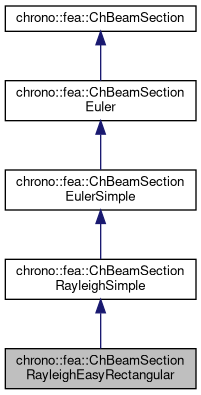chrono::fea::ChBeamSectionRayleighEasyRectangular Class Reference

## Description

This works exactly as ChBeamSectionEulerEasyRectangular, but adds the effect of Jyy Jzz rotational sectional inertias.

#include <ChBeamSectionEuler.h>

Inheritance diagram for chrono::fea::ChBeamSectionRayleighEasyRectangular:[legend]
Collaboration diagram for chrono::fea::ChBeamSectionRayleighEasyRectangular:[legend]

## Public Member Functions

ChBeamSectionRayleighEasyRectangular (double width_y, double width_z, double E, double G, double density)Public Member Functions inherited from chrono::fea::ChBeamSectionRayleighSimple
virtual void ComputeInertiaMatrix (ChMatrixNM< double, 6, 6 > &M) override
Compute the 6x6 sectional inertia matrix, as in {x_momentum,w_momentum}=[Mm]{xvel,wvel}.Public Member Functions inherited from chrono::fea::ChBeamSectionEulerSimple
void SetArea (const double ma)
Set the cross sectional area A of the beam (m^2)

double GetArea () const

void SetIyy (double ma)
Set the Iyy moment of inertia of the beam (for flexion about y axis) Note: some textbook calls this Iyy as Iz.

double GetIyy () const

void SetIzz (double ma)
Set the Izz moment of inertia of the beam (for flexion about z axis) Note: some textbook calls this Izz as Iy.

double GetIzz () const

void SetJ (double ma)
Set the J torsion constant of the beam (for torsion about x axis)

double GetJ () const

void SetKsy (double ma)
Set the Timoshenko shear coefficient Ks for y shear, usually about 0.8, (for elements that use this, ex. More...

double GetKsy () const

void SetKsz (double ma)
Set the Timoshenko shear coefficient Ks for z shear, usually about 0.8, (for elements that use this, ex. More...

double GetKsz () const

void SetAsRectangularSection (double width_y, double width_z)
Shortcut: set Area, Ixx, Iyy, Ksy, Ksz and J torsion constant at once, given the y and z widths of the beam assumed with rectangular shape.

void SetAsCircularSection (double diameter)
Shortcut: set Area, Ixx, Iyy, Ksy, Ksz and J torsion constant at once, given the diameter of the beam assumed with circular shape.

void SetDensity (double md)
Set the density of the beam (kg/m^3)

double GetDensity () const

void SetYoungModulus (double mE)
Set E, the Young elastic modulus (N/m^2)

double GetYoungModulus () const

void SetGshearModulus (double mG)
Set G, the shear modulus, used for computing the torsion rigidity = J*G.

double GetGshearModulus () const

void SetGwithPoissonRatio (double mpoisson)
Set G, the shear modulus, given current E and the specified Poisson ratio.

virtual double GetAxialRigidity () const override
Gets the axial rigidity, usually A*E, but might be ad hoc.

virtual double GetXtorsionRigidity () const override
Gets the torsion rigidity, for torsion about X axis at elastic center, usually J*G, but might be ad hoc.

virtual double GetYbendingRigidity () const override
Gets the bending rigidity, for bending about Y axis at elastic center, usually Iyy*E, but might be ad hoc.

virtual double GetZbendingRigidity () const override
Gets the bending rigidity, for bending about Z axis at elastic center, usually Izz*E, but might be ad hoc.

virtual double GetSectionRotation () const override
Set the rotation of the Y Z section axes for which the YbendingRigidity and ZbendingRigidity are defined.

virtual double GetCentroidY () const override
Gets the Y position of the elastic center respect to centerline.

virtual double GetCentroidZ () const override
Gets the Z position of the elastic center respect to centerline.

virtual double GetShearCenterY () const override
Gets the Y position of the shear center respect to centerline.

virtual double GetShearCenterZ () const override
Gets the Z position of the shear center respect to centerline.

virtual void ComputeQuadraticTerms (ChVector<> &mF, ChVector<> &mT, const ChVector<> &mW) override
Compute the centrifugal term and gyroscopic term.

virtual double GetMassPerUnitLength () const override
Get mass per unit length, ex.SI units [kg/m].

virtual double GetInertiaJxxPerUnitLength () const override
Get the Jxx component of the inertia per unit length (polar inertia) in the Y Z unrotated reference frame of the section at centerline. More...Public Member Functions inherited from chrono::fea::ChBeamSectionEuler
void SetArtificialJyyJzzFactor (double mf)
The Euler beam model has no rotational inertia per each section, assuming mass is concentrated on the centerline. More...

double GetArtificialJyyJzzFactor ()

void SetBeamRaleyghDamping (double mr)
Set the Rayleigh damping ratio r (as in: R = r * K ), to do: also mass-proportional term.

double GetBeamRaleyghDamping ()Public Member Functions inherited from chrono::fea::ChBeamSection
void SetDrawShape (std::shared_ptr< ChBeamSectionShape > mshape)
Set the graphical representation for this section. More...

std::shared_ptr< ChBeamSectionShapeGetDrawShape () const
Get the drawing shape of this section (i.e.a 2D profile used for drawing 3D tesselation and visualization) By default a thin square section, use SetDrawShape() to change it.

void SetDrawThickness (double thickness_y, double thickness_z)
Shortcut: adds a ChBeamSectionShapeRectangular for visualization as a centered rectangular beam, and sets its width/height. More...

Shortcut: adds a ChBeamSectionShapeCircular for visualization as a centered circular beam, and sets its radius. More...

void SetCircular (bool ic)
OBSOLETE only for backward compability More...Public Attributes inherited from chrono::fea::ChBeamSectionEulerSimple
double Area

double Iyy

double Izz

double J

double G

double E

double density

double Ks_y

double Ks_zProtected Attributes inherited from chrono::fea::ChBeamSectionEuler
double JzzJyy_factor

## ◆ ChBeamSectionRayleighEasyRectangular()

 chrono::fea::ChBeamSectionRayleighEasyRectangular::ChBeamSectionRayleighEasyRectangular ( double width_y, double width_z, double E, double G, double density )
Parameters
 width_y width of section in y direction width_z width of section in z direction E Young modulus G Shear modulus (only needed for the torsion) density volumetric density (ex. in SI units: [kg/m^3])

The documentation for this class was generated from the following files:
• /builds/uwsbel/chrono/src/chrono/fea/ChBeamSectionEuler.h
• /builds/uwsbel/chrono/src/chrono/fea/ChBeamSectionEuler.cpp